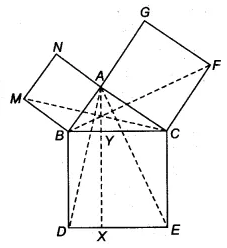Q

# In Fig. 9 34, ABC is a right triangle right angled at A. BCED, ACFG and ABMN are squares on the sides BC, CA and AB respectively. Line segment AX perpendicular to DE meets BC at Y. Show that: (ii)

Q : 8     In Fig. , ABC is a right triangle right angled at A. BCED, ACFG and ABMN are squares on the sides BC, CA and AB respectively. Line segment   meets BC at Y. Show that:

(ii)ViewsSInce ||gm BYXD and ABD are on the same base BD and between same parallels BD and AX
Therefore, ar(ABD) = 1/2. ar(||gm BYXD)..........(i)

But, ABD  MBC (proved in 1st part)
Since congruent triangles have equal areas.
Therefore, By using equation (i) we get

Hence proved

Exams
Articles
Questions